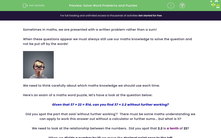# Solve Word Problems and Puzzles

In this worksheet, students resolve word problems and puzzles allowing them to revise and practise a range of mathematical processes for measures, percentages, decimals, fractions and wider number knowlege.Key stage:  KS 3

Curriculum topic:   Number

Curriculum subtopic:   Use Four Operations for All Numbers

Difficulty level:#### Worksheet Overview

Sometimes in maths, we are presented with a written problem rather than a sum!

When these questions appear we must always still use our maths knowledge to solve the question and not be put off by the words!We need to think carefully about which maths knowledge we should use each time.

Here's an exam of a maths word puzzle, let's have a look at the question below:

Given that 37 × 22 = 814, can you find 37 × 2.2 without further working?

Did you spot the part that said 'without further working'?  There must be some maths understanding we can apply to work this answer out without a calculator or further sums... but what is it?

We need to look at the relationship between the numbers.  Did you spot that 2.2 is a tenth of 22?

When we divide a number by 10 we move the decimal point once to the left

37 × 2.2 = 814 ÷ 10 = 81.4

Let's give this a go!

### What is EdPlace?

We're your National Curriculum aligned online education content provider helping each child succeed in English, maths and science from year 1 to GCSE. With an EdPlace account you’ll be able to track and measure progress, helping each child achieve their best. We build confidence and attainment by personalising each child’s learning at a level that suits them.

Get started